# Tail Recursion

## Tail Recursion with Examples

In this article, I am going to discuss Tail Recursion in C with Examples. Please read our previous article where we discussed Static and Global Variables in Recursion. At the end of this article, you will understand the following pointers in detail.

1. Types of Recursion
2. What is Tail Recursion?
3. Tail Recursion Examples.
4. How to Convert Tail Recursion to a loop?
5. Which one to choose between Tail Recursion and Loop?
##### Types of Recursion:

There are five types of recursions. They are as follows:

1. Tail Recursion
3. Tree Recursion
4. Indirect Recursion
5. Nested Recursion

Note: We will discuss each of the above recursion with examples as well as we will also see the differences between. Also, we will try to compare the recursion with the loop and see the time and complexity, and then we will make the decision whether we need to use the Recursive function or should we go with looping.

##### Tail Recursion in C:

We have already seen the example of tail recursion in our previous articles. The following is an example of Tail Recursion.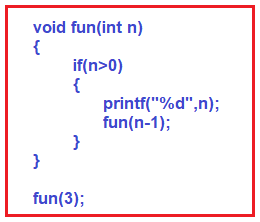##### What does it mean by Tail Recursion?

If a function is calling itself and that recursive call is the last statement in a function then it is called tail recursion. After that call there is nothing, it is not performing anything, so, it is called tail recursion. For better understanding, please have a look at the below image.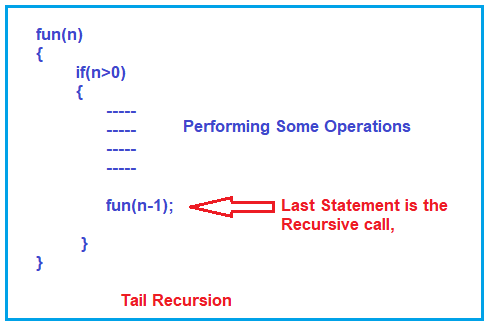As you can see in the above image, the fun function taking some parameter ‘n’ and if n>0, then there are some statements inside the if block, and further if you notice the last statement it is calling itself by a reduced value of n. So. what all it has to do; it is performing the operations first and then it is calling itself.

So, the point that you need to remember is, if the last statement is a recursive function call then it is called tail recursion. This also means that all the operations will perform at calling time only and the function will not be performing any operation at a returning time. Everything will be performed at calling time only and that is why it is called tail recursion.

##### Example: Tail Recursion in C Language

The following is an example of Tail Recursion. As you can see, there is nothing, there is no operation we are performing after the recursive call and that recursive function call is the last statement.

```#include <stdio.h>
void fun(int n)
{
if (n > 0)
{
printf("%d", n);
fun(n-1);
}
}

int main ()
{
fun(3);
return 0;
}
```

Output: 321

###### The following example is not Tail Recursion.

As you can see in the below example, there is something written (+n) along with the function call i.e. some operation is going to be performed at the returning time. So, in this function, there is something remaining that has to be performed at returning time and hence cannot be tail recursion. Tail recursion means at returning time it doesn’t have to perform anything at all.

```#include <stdio.h>
void fun(int n)
{
if (n > 0)
{
printf("%d", n);
fun(n-1) + n;
}
}

int main ()
{
fun(3);
return 0;
}
```
##### Tail Recursion vs Loops in C:

Now, we will compare tail recursion with loops. The first and the foremost thing that we need to remember is every recursive function can be written using a loop and vice versa is also true i.e. every loop can be written using a recursive function.

The following is an example of Tail Recursive that we have just discussed. Already in our previous article, we traced this function and know that the output will be 321 when we pass the value 3 to the function.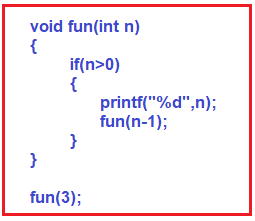Now we want to write the above recursive function using a loop. The following image shows how to convert the recursive function to a loop. Here, instead of the conditional if statement, we use a while loop, and instead of the recursive function call with a reduced value of n, we directly reduced the n value by 1.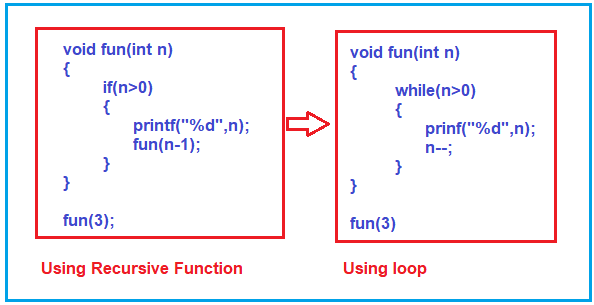##### Example: Using Loop

The following example uses a loop and gets the same output as the recursive function. If you call the fun function bypassing the value 3, then you will also get the same output 321 as we get in the Recursive function example.

```#include <stdio.h>
void fun(int n)
{
while (n > 0)
{
printf("%d", n);
n--;
}
}

int main ()
{
fun(3);
return 0;
}
```

Output: 321

The output is the same as well as the structure also looks similar between recursive function and loop. So, the point that I have to tell you here is that tail recursion can be easily converted in the form of a loop.

##### Which one to choose between Tail Recursive and Loop?

Let us decide which one is efficient. For this, we are going to compare the two examples that we have already discussed in this article. Please have a look at the below image.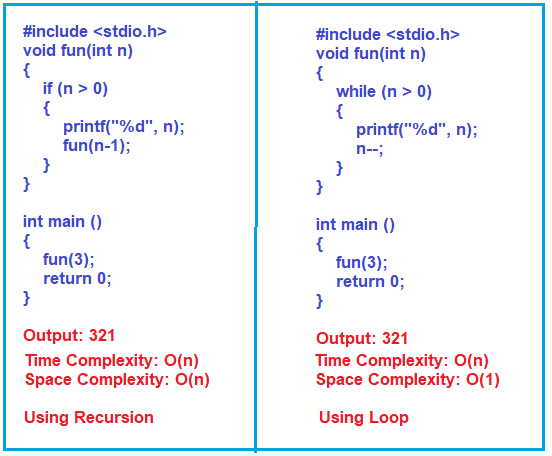###### Time Complexity:

In terms of time Complexity, if you analyze, both the function printing the same three values. That means the amount of time spent is the same whatever the value of ‘n’ is given. So, the time taken by both of them is the order of n i.e. O(n).

###### Space Complexity:

The recursive function internally utilizes a stack. For the value of 3, it will create a total of 4 activation records in a stack. Already we have done the analysis in our previous article. So, for a value n, the space taken by the Recursive mechanism is the order of n i.e. O(n)

But in the loop, only 1 activation record will be created as it is not calling itself again. So, the space complexity of the loop is the order of 1 i.e. O(1) and it will just create only one activation record that is constant.

So, the conclusion is, if you have to write a tail recursion then better convert it into a loop that is more efficient in terms of space. But this will not be true for every type of recursion or loop. So, in the case of Tail Recursion, the loop is efficient.

Note: One more point that you have to remember is some compilers (under code optimization inside compiler), will check, if you have written any function which is tail recursion, then they will try to convert it in the form of a loop. It means they will try to reduce the space consumption and they will utilize only the order of 1 i.e. O(1).

In the next article, I am going to discuss Head Recursion with Examples. Here, in this article, I try to explain Tail Recursion in C with Examples and I hope you enjoy this Tail Recursion in C with Examples article.